# 最小二乘法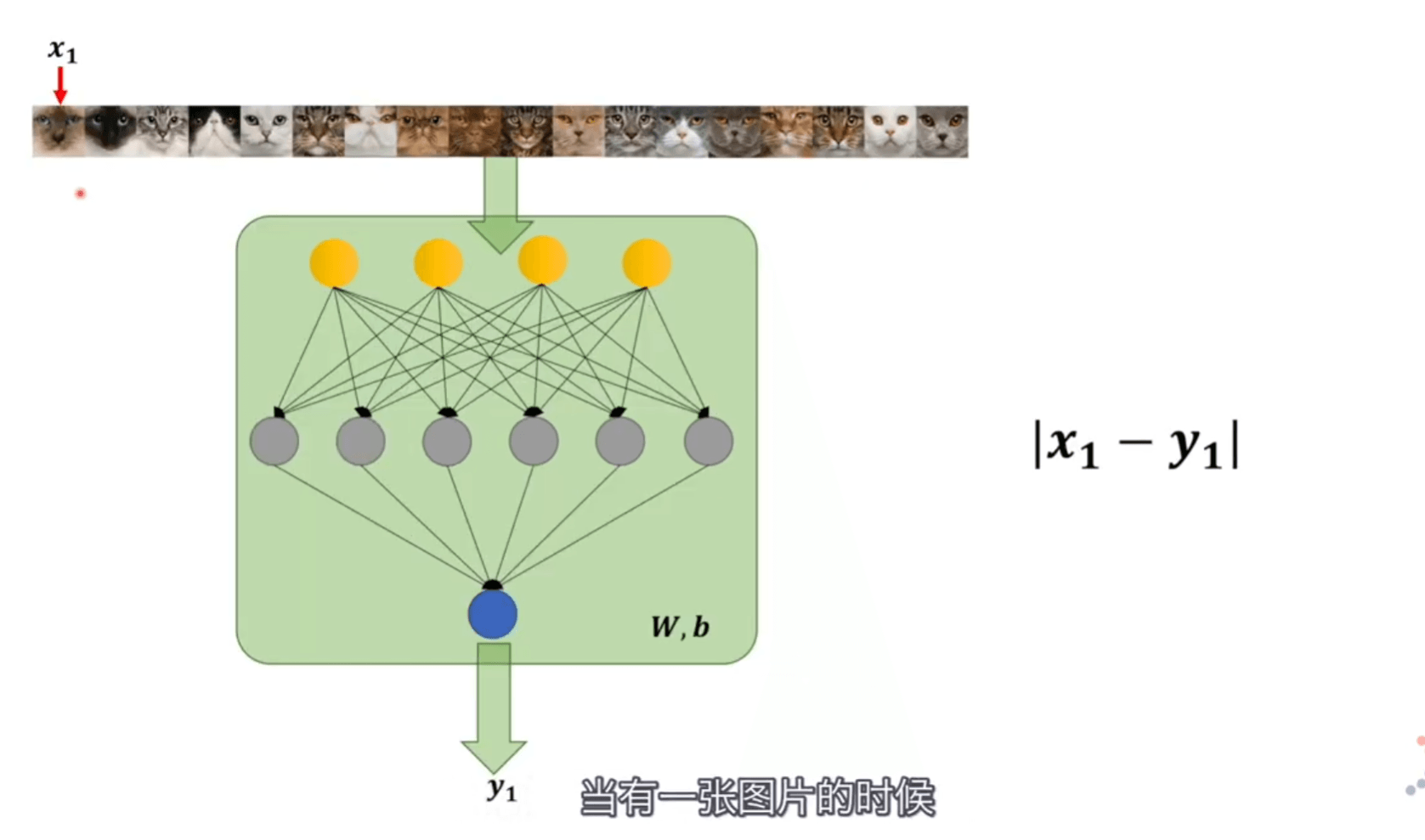# 极大似然估计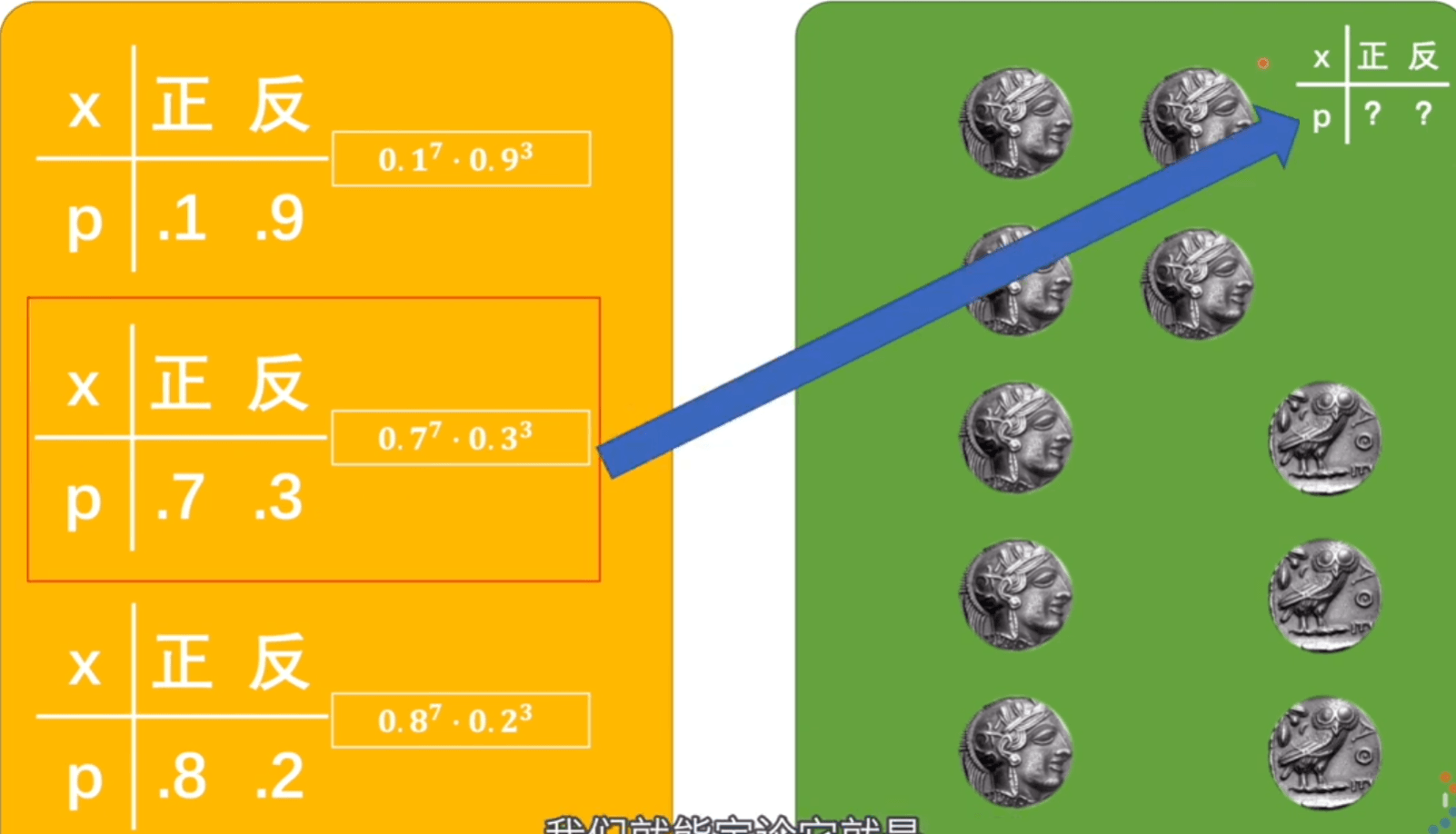$\begin{array}{l} P\left(C_{1}, C_{2}, C_{3}, \ldots, C_{10} \mid \theta\right) \\ P\left(x_{1}, x_{2}, x_{3}, x_{4}, \ldots, x_{n} \mid W, b\right) \end{array}$

$\theta$ 是抛硬币的概率模型，$W,b$ 是神经网络的概率模型。前者结果是硬币是正还是反，后者结果是图片到底是不是猫。

$\begin{array}{l} P\left(x_{1}, x_{2}, x_{3}, x_{4}, \ldots, x_{n} \mid W, b\right) \\ =\prod_{i=1}^{n} P\left(x_{i} \mid W, b\right) \end{array}$

$\begin{array}{l} =\prod_{i=1}^{n} P\left(x_{i} \mid W, b\right) \\ =\prod_{i=1}^{n} P\left(x_{i} \mid y_{i}\right) \end{array}$

$x_{i}$ 的取值是 $0、1$ ，符合二项伯努利分布，概率分布表达式为

$f(x)=p^{x}(1-p)^{1-x}=\left\{\begin{array}{ll} p, & x=1 \\ 1-p, & x=0 \end{array}\right.$

$x=1$ 就是图片为猫的概率。而 $p$ 就是 $y_{i}$ (神经网络认定是猫的概率)，将其带入替换 $P\left(x_{i} \mid y_{i}\right)$

$=\prod_{i=1}^{n} y_{i}^{x_{i}}\left(1-y_{i}\right)^{1-x_{i}}$

$\begin{array}{l} \log \left(\prod_{i=1}^{n} y_{i}^{x_{i}}\left(1-y_{i}\right)^{1-x_{i}}\right) \\ =\sum_{i=1}^{n} \log \left(y_{i}^{x_{i}}\left(1-y_{i}\right)^{1-x_{i}}\right) \\ =\sum_{i=1}^{n}\left(x_{i} \cdot \log y_{i}+\left(1-x_{i}\right) \cdot \log \left(1-y_{i}\right)\right) \end{array}$

$\begin{array}{l} \max \left(\sum_{i=1}^{n}\left(x_{i} \cdot \log y_{i}+\left(1-x_{i}\right) \cdot \log \left(1-y_{i}\right)\right)\right) \\ \min -\left(\sum_{i=1}^{n}\left(x_{i} \cdot \log y_{i}+\left(1-x_{i}\right) \cdot \log \left(1-y_{i}\right)\right)\right) \end{array}$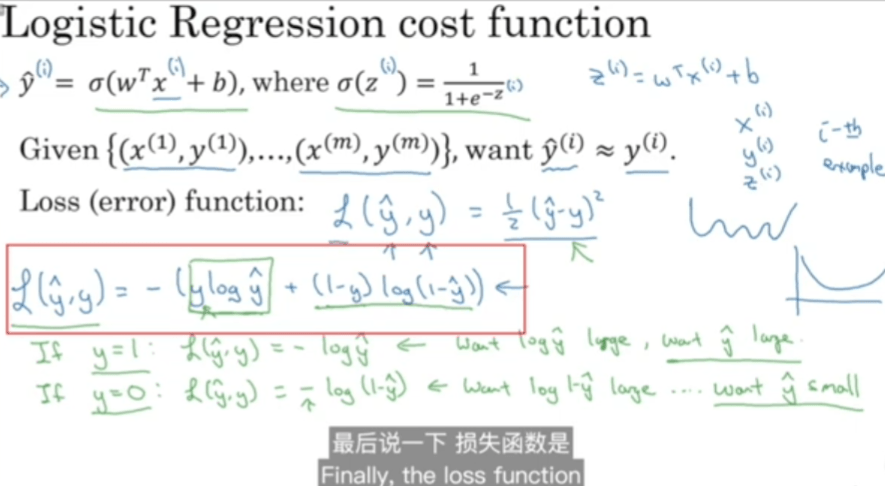### 复习一下对数

1. $\log _{a}(1)=0$
2. $\log _{a}(a)=1$
3. $负数与零无对数$
4. $\log _{a} b * \log _{b} a=1$
5. $\log _{a}(M N)=\log _{a} M+\log _{a} N$
6. $\log _{a}(M / N)=\log _{a} M-\log _{a} N$
7. $\log _{a} M^{n}=n \log _{a} M(\mathrm{M}, \mathrm{N} \in \mathrm{R})$
8. $\log _{a^{n}} M=\frac{1}{n} \log _{a} M$
9. $a^{\log _{a} b}=b$

# 交叉熵

## 信息量

${f}({x}):=\text { 信息量 }\\ {f}( 阿根廷夺冠 )={f}( 阿根廷进决赛 )+{f}( 阿根廷赢了决赛 ) \\ f\left(\frac{1}{8}\right)=f\left(\frac{1}{4}\right)+f\left(\frac{1}{2}\right)\\ P(\text { 阿根廷夺冠 })=P(\text { 阿根廷进决赛 }) \cdot {P} \text { (阿根廷赢了决赛 })\\$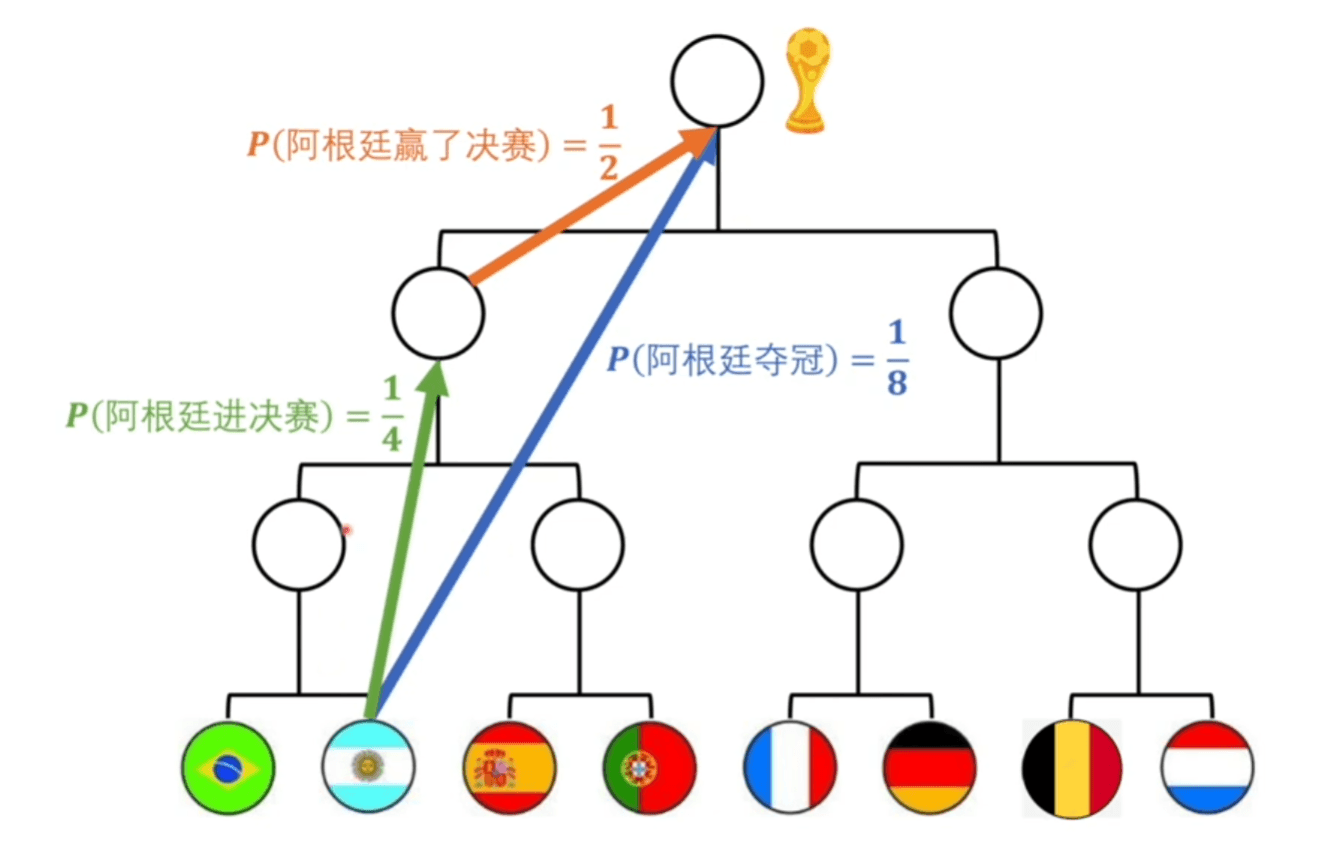$\begin{array}{c} f(x):=? \log _{?} x \\ f\left(x_{1} \cdot x_{2}\right)=f\left(x_{1}\right)+f\left(x_{2}\right) \end{array}$

$\begin{array}{c} f(x):=-\log _{2} x \\ f\left(x_{1} \cdot x_{2}\right)=f\left(x_{1}\right)+f\left(x_{2}\right) \end{array}$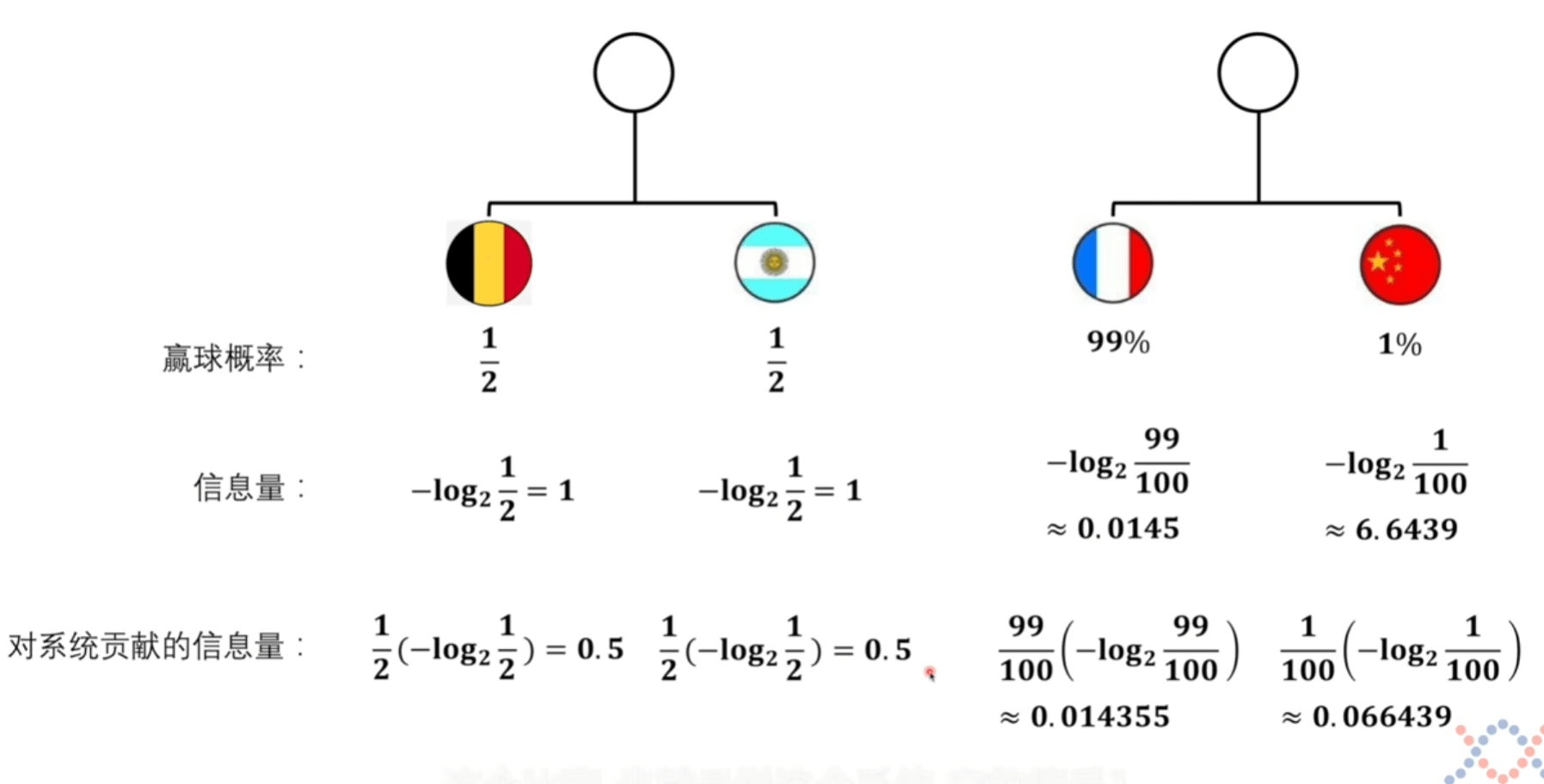## 系统熵的定义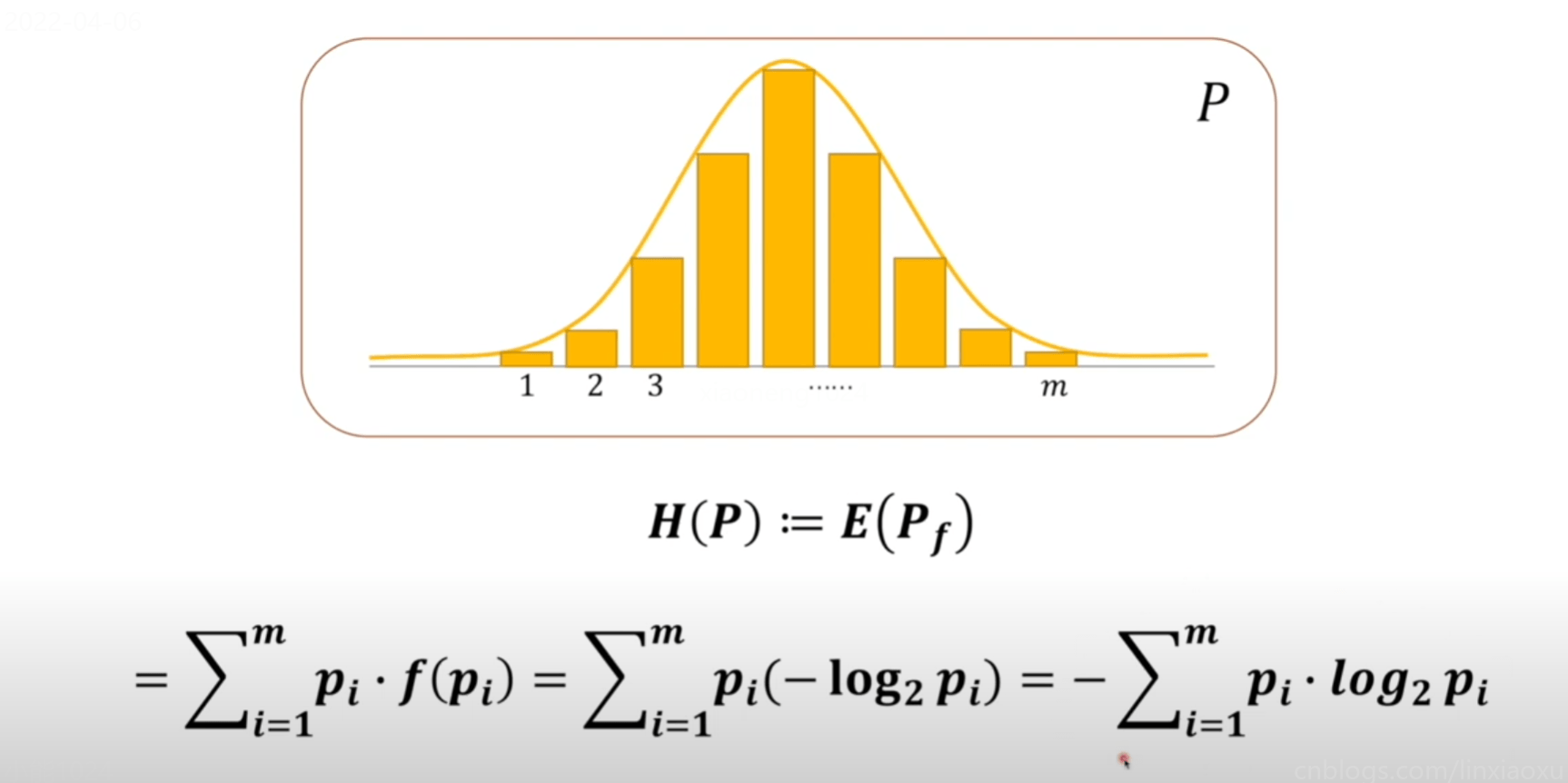## KL散度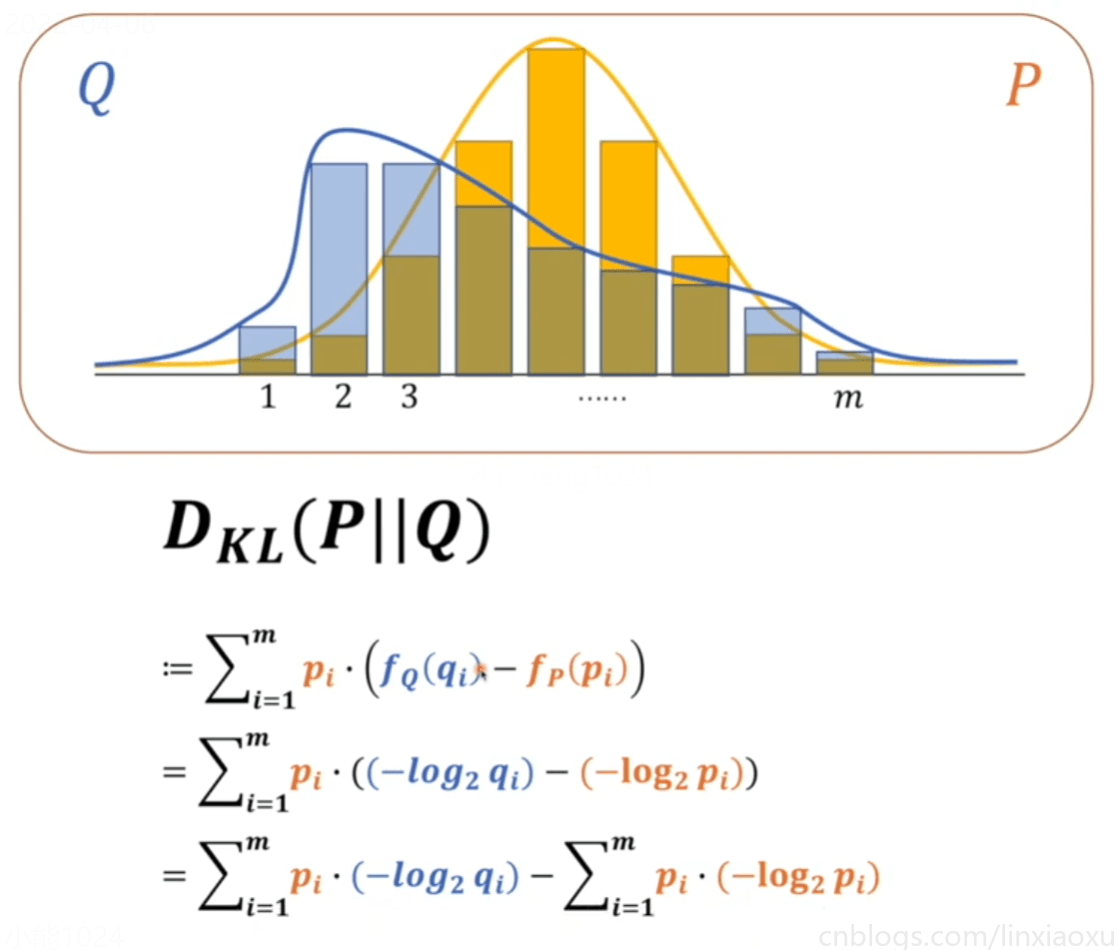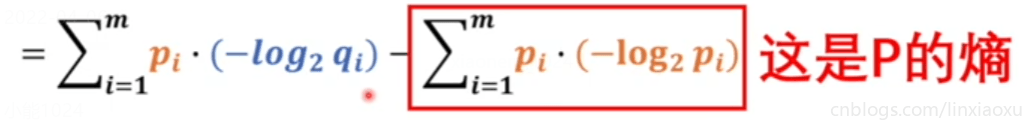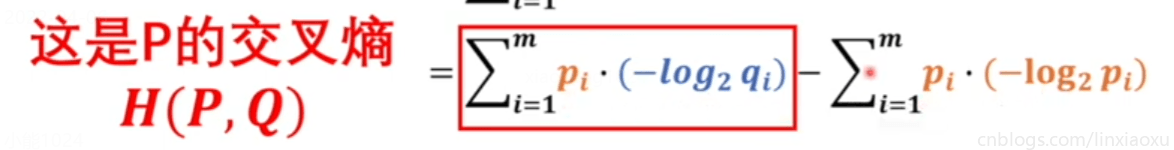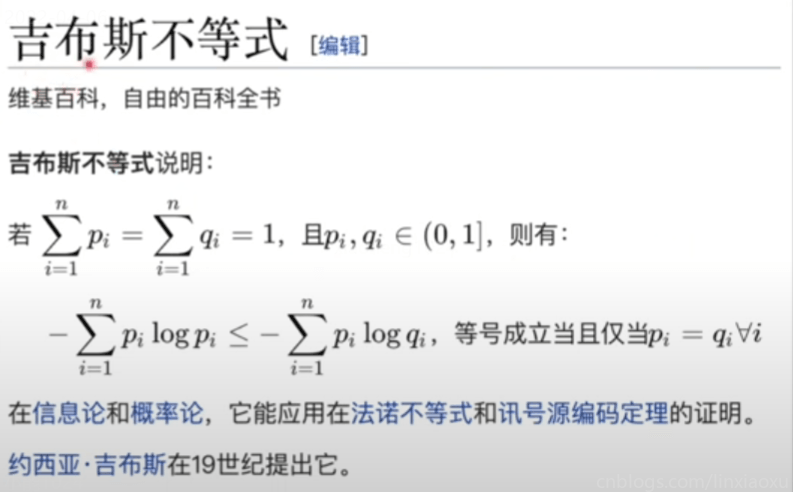KL散度绝对是大于等于$0$的，当$Q、P$相等的时候等于$0$，不相等的时候一定大于$0$

$m$选择的解释：假如$p$的事件数量是$m$$q$的事件数量是$n$$m＞n$，那么写成$∑$求和，用较大的$m$做上标。就可以分解为，$∑1到n+∑n+1到m$，那么对于$q$来说，因为$q$的数量只有$n$，那么对应的$q$的部分$∑n+1到m$都等于$0$

$\begin{array}{l} \boldsymbol{H}(\boldsymbol{P}, \boldsymbol{Q}) \\ =\sum_{i=1}^{m} p_{i} \cdot\left(-\log _{2} q_{i}\right) \\ =\sum_{i=1}^{n} x_{i} \cdot\left(-\log _{2} q_{i}\right) \\ =-\sum_{i=1}^{n}\left(x_{i} \cdot \log _{2} y_{i}+\left(1-x_{i}\right) \cdot \log _{2}\left(1-y_{i}\right)\right) \end{array}$

$P$ 是基准，要被比较的概率模型，我们要比较的人脑模型，要么完全是猫要么不是猫。

$x_{i}$ 有两种情况，而 $y_{i}$ 只判断图片有多像猫，并没有去判断相反的这个猫有多不像猫，而公式里的 $x_{i}$$q_{i}$ 要对应起来，当 $x_{i}$$1$ ，要判断多像猫，当 $x_{i}$$0$ 的时候，要判断不像猫的概率。

• 极大似然法里的 $log$ 使我们按习惯引入的，把连乘换成相加。而交叉熵的 $log$ 是写在信息量定义里的，以 $2$ 为底，计算出来的单位是比特，是有量纲的。
• 极大似然法求的是最大值，我们按习惯求最小值。而交叉熵负号是写在定义里的。
posted @ 2022-04-07 11:17  小能日记  阅读(26)  评论(0编辑  收藏  举报